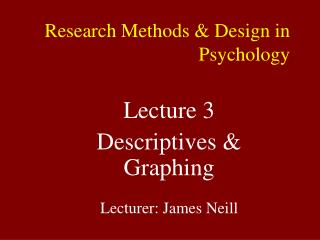DownloadDownload PresentationTélécharger la présentation- - - - - - - - - - - - - - - - - - - - - - - - - - - E N D - - - - - - - - - - - - - - - - - - - - - - - - - - -
##### Presentation Transcript

1. Lecture 3 Descriptives & Graphing Lecturer: James Neill Research Methods & Design in Psychology

2. Overview Univariate descriptives & graphs Non-parametric vs. parametric Non-normal distributions Properties of normal distributions Graphing relations b/w 2 and 3 variables

3. Empirical Approach to Research A positivistic approach ASSUMES: the world is made up of bits of data which can be ‘measured’, ‘recorded’, & ‘analysed’ Interpretation of data can lead to valid insights about how people think, feel and behave

4. What do we want to Describe? Distributional properties of variables: Central tendency(ies) Shape Spread / Dispersion

5. Basic Univariate Descriptive Statistics Central tendency Mode Median Mean Spread • Interquartile Range • Range • Standard Deviation • Variance Shape • Skewness • Kurtosis

6. Basic Univariate Graphs Bar Graph – Pie Chart Stem & Leaf Plot Boxplot Histogram

7. Measures of Central Tendency Statistics to represent the ‘centre’ of a distribution Mode (most frequent) Median (50th percentile) Mean (average) Choice of measure dependent on Type of data Shape of distribution (esp. skewness)

8. Measures of Central Tendency Mode Median Mean Nominal X Ordinal X X Interval X X X Ratio X? X X

9. Measures of Dispersion Measures of deviation from the central tendency Non-parametric / non-normal:range, percentiles, min, max Parametric:SD & properties of the normal distribution

10. Measures of Dispersion Range, Min/Max Percentiles SD Nominal Ordinal X Interval X X X? Ratio X X X

11. Describing Nominal Data Frequencies Most frequent? Least frequent? Percentages? Bar graphs Examine comparative heights of bars – shape is arbitrary Consider whether to use freqs or %s

12. Frequencies Number of individuals obtaining each score on a variable Frequency tables graphically (bar chart, pie chart) Can also present as %

13. Frequency table for sex

14. Bar chart for frequency by sex

15. Pie chart for frequency by sex

16. Bar chart: Do you believe in God?

17. Bar chart for cost by state

18. Bar chart vs. Radar Chart

19. Bar chart vs. Radar Chart

20. Mode Most common score - highest point in a distribution Suitable for all types of data including nominal (may not be useful for ratio) Before using, check frequencies and bar graph to see whether it is an accurate and useful statistic.

21. Describing Ordinal Data Conveys order but not distance (e.g., ranks) Descriptives as for nominal (i.e., frequencies, mode) Also maybe median – if accurate/useful Maybe IQR, min. & max. Bar graphs, pie charts, & stem-&-leaf plots

22. Stem & Leaf Plot • Useful for ordinal, interval and ratio data • Alternative to histogram

23. Box & whisker • Useful for interval and ratio data • Represents min. max, median and quartiles

24. Describing Interval Data Conveys order and distance, but no true zero (0 pt is arbitrary). Interval data is discrete, but is often treated as ratio/continuous (especially for > 5 intervals) Distribution (shape) Central tendency (mode, median) Dispersion (min, max, range) Can also use M & SD if treating as continuous

25. Describing Ratio Data Numbers convey order and distance, true zero point - can talk meaningfully about ratios. Continuous Distribution (shape – skewness, kurtosis) Central tendency (median, mean) Dispersion (min, max, range, SD)

26. Univariate data plot for a ratio variable

27. The Four Moments of a Normal Distribution Mean <-Kurt-> <-SD-> <-Skew Skew->

28. The Four Moments of a Normal Distribution Four mathematical qualities (parameters) allow one to describe a continuous distribution which as least roughly follows a bell curve shape: • 1st = mean (central tendency) • 2nd = SD (dispersion) • 3rd = skewness (lean / tail) • 4th = kurtosis (peakedness / flattness)

29. Mean (1st moment ) • Average score • Mean =  X / N • Use for ratio data or interval (if treating it as continuous). • Influenced by extreme scores (outliers)

30. Standard Deviation (2nd moment ) • SD = square root of Variance =  (X - X)2 N – 1 • Standard Error (SE) = SD / square root of N

31. Skewness (3rd moment ) • Lean of distribution • +ve = tail to right • -ve = tail to left • Can be caused by an outlier • Can be caused by ceiling or floor effects • Can be accurate (e.g., the number of cars owned per person)

32. Skewness (3rd moment ) • Negative skew • Positive skew

33. Ceiling Effect

34. Floor Effect

35. Kurtosis (4th moment ) • Flatness or peakedness of distribution • +ve = peaked • -ve = flattened • Be aware that by altering the X and Y axis, any distribution can be made to look more peaked or more flat – so add a normal curve to the histogram to help judge kurtosis

36. Kurtosis (4th moment ) Red = Positive (leptokurtic) Blue = negative (platykurtic)

37. Key Areas under the Curve for Normal Distributions • For normal distributions, approx. +/- 1 SD = 68%+/- 2 SD ~ 95%+/- 3 SD ~ 99.9%

38. Areas under the normal curve

39. Types of Non-normal Distribution • Bi-modal • Multi-modal • Positively skewed • Negatively skewed • Flat (platykurtic) • Peaked (leptokurtic)

40. Non-normal distributions

41. Non-normal distributions

42. Rules of Thumb in Judging Severity of Skewness & Kurtosis • View histogram with normal curve • Deal with outliers • Skewness / kurtosis <-1 or >1 • Skewness / kurtosis significance tests

43. Histogram of weight

44. Histogram of daily calorie intake

45. Histogram of fertility

46. Example ‘normal’ distribution 1

47. Example ‘normal’ distribution 2

48. Example ‘normal’ distribution 3

49. Example ‘normal’ distribution 4

50. Example ‘normal’ distribution 5